# Precalculus : Determine the equation of a parabola and graph a parabola

## Example Questions

1 2 3 5 Next →

### Example Question #55 : Parabolas

Find the focus of the parabola with the following equation: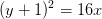Possible Answers: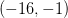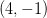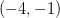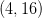Correct answer:Explanation:

Recall the standard form of the equation of a horizontal parabola: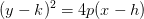, where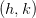is the vertex of the parabola and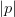is the focal length.

When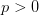, the parabola opens to the right.

When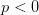, the parabola opens to the left.

For the given parabola, the vertex is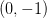and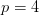. This means the parabola is opening to the right and that the focus will be locatedunits to the right of the vertex. The focus is then located at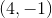.

### Example Question #56 : Parabolas

Find the focus of the parabola with the following equation: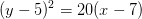Possible Answers: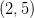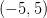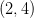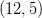Correct answer:Explanation:

Recall the standard form of the equation of a horizontal parabola:, whereis the vertex of the parabola andis the focal length.

When, the parabola opens to the right.

When, the parabola opens to the left.

For the given parabola, the vertex is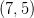and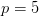. This means the parabola is opening to the right and that the focus will be located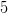units to the right of the vertex. The focus is then located at.

### Example Question #57 : Parabolas

Find the focus of the parabola with the following equation: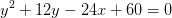Possible Answers: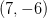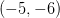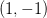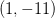Correct answer:Explanation:

Recall the standard form of the equation of a horizontal parabola:, whereis the vertex of the parabola andis the focal length.

When, the parabola opens to the right.

When, the parabola opens to the left.

Start by putting the equation into the standard form of the equation of a horizontal parabola.

Isolate theterms on one side.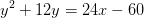Complete the square. Remember to add the same amount to both sides of the equation!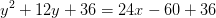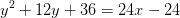Factor both sides of the equation to get the equation in the standard form.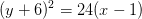For the given parabola, the vertex is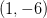and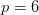. This means the parabola is opening to the right and that the focus will be locatedunits to the right of the vertex. The focus is then located at.

### Example Question #58 : Parabolas

Find the focus and directrix of the parabola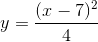.

Possible Answers:

Focus: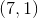; Directrix: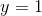Focus: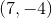; Directrix: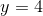Focus:; Directrix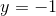Focus: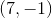; Directrix:Focus: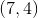; Directrix:Correct answer:

Focus:; DirectrixExplanation:

We can first re-write the equation by multiplying both sides by 4: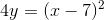This equation tells us that the vertex for the parabola is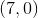We can also find the distance from the vertex to the focus and the directrix by setting up the equation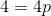. This gives us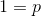, so the focus and the directrix are both 1 away from the vertex.

Since this equation is positive, the parabola opens up. This means the directrix is one below the vertex, so it is at. The focus is one above the vertex, so it is at.

### Example Question #59 : Parabolas

Find the focus and directrix of the parabola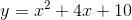.

Possible Answers:

Focus: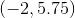; Directrix: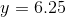Focus: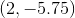; Directrix: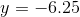Focus: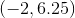; Directrix: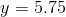Focus: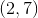; Directrix: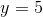Focus: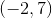; Directrix:Correct answer:

Focus:; Directrix:Explanation:

Before we can start determining the focus and directrix, we need to re-write this equation in standard form. That way we can see the vertex and we can more easily determine the distance from the vertex and the focus/directrix.

To do this, we should complete the square. First subtract 10 from both sides: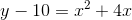Now we need to figure out what to add to both sides in order to make the right side a perfect square. We should add 4, since half of the 4 in 4x is 2, and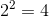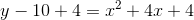We can simplify the left side and re-write the right side as a binomial squared: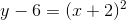Interpreting this, we determine that the vertex is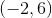. On the left side,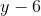is multiplied by 1, so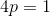where p measures the distance from the vertex to the focus/directrix. Solving this gives us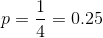Since this is a positive, upward-opening parabola, the directrix is below the vertex and the focus is above. The focus is then located at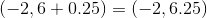. The directrix is then located at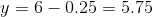.

### Example Question #60 : Parabolas

Determine the direction in which the following parabola opens.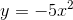Possible Answers:

Downwards

Upwards

Leftwards

Rightwards

Correct answer:

Downwards

Explanation:

For the function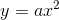The parabola opens upwards if a>0

and downards for a<0

Because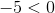The parabola opens downwards.

1 2 3 5 Next →

### All Precalculus Resources